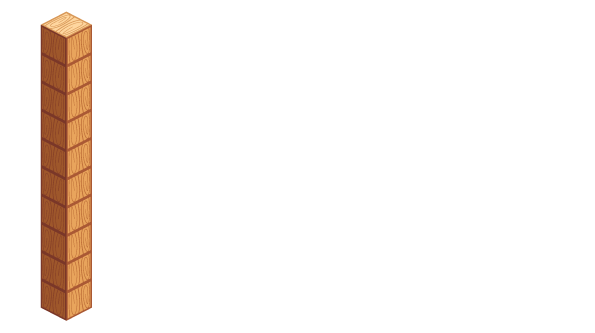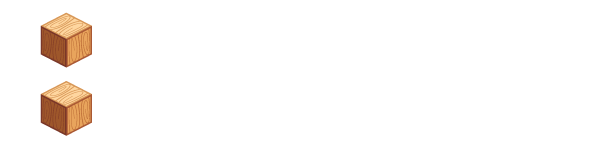Place value is the value of each digit in a number.

Let's look at the number 12.

This block represents the number 10.There are 10 cubes in the block.

These single blocks represent units or ones.There are 2 cubes.

If we add one 10 and two 1s together, we have 12.

10 + 2 = 12